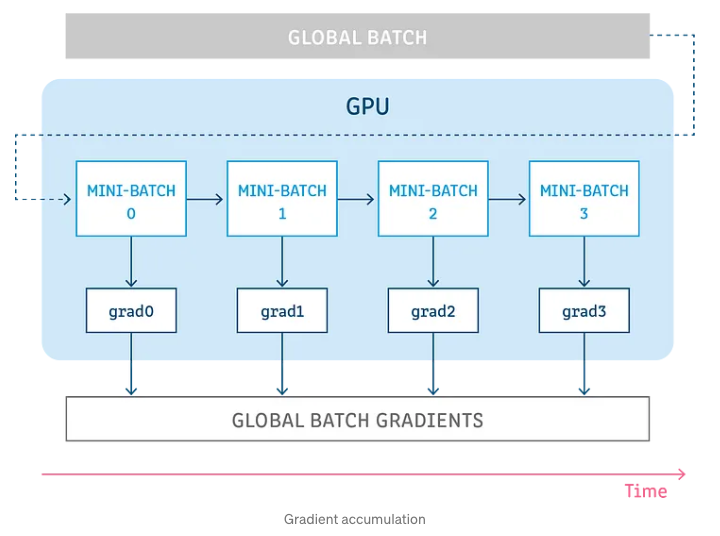## Overview

• With models getting larger, running out of GPU memory and getting a ‘RuntimeError’: CUDA error: out of memory error has become more ubiquitous.
• Here, we will talk about a few ways to optimize your models performance and allow it to scale by saving the GPU memory.

• Gradient accumulation is a technique used in deep learning to increase the effective batch size during training. Normally, the weights of a neural network are updated based on the gradients computed from a single batch of training data. However, for larger models or datasets, the batch size may be limited by the memory capacity of the GPU.
• As shown in the image below, gradient accumulation splits the batch of samples (that are used to train a neural network) into several mini batches that are run sequentially.• “The idea behind gradient accumulation is to instead of calculating the gradients for the whole batch at once to do it in smaller steps. The way we do that is to calculate the gradients iteratively in smaller batches by doing a forward and backward pass through the model and accumulating the gradients in the process.” (source)
• Once we have enough gradients accumulated via the above process, we run the model’s optimization step to increase the overall batch size.
• The code sample below shows how the model gets impacted positively by gradient accumulation since without it, the model had a time of 57.82, samples per second of 8.86 and GPU memory of 14949 MB.
training_args = TrainingArguments(per_device_train_batch_size=1, gradient_accumulation_steps=4, **default_args)

trainer = Trainer(model=model, args=training_args, train_dataset=ds)
result = trainer.train()
print_summary(result)

Time: 66.03
Samples/second: 7.75
GPU memory occupied: 8681 MB.

• Gradient accumulation can lead to slower convergence and longer training times, as the gradients are accumulated over several mini-batches before an update is made. However, it can be a useful technique in situations where memory is limited, and a larger effective batch size is desired.
• The code below helps illustrate the basic idea behind gradient accumulation. In it, we train a loop of ‘num_iterations’ iterations and within each iteration, ‘accumulation_step’ mini-batches are processed before updating the weights.
• During each iteration, the gradients for each mini-batch are computed separately using compute_gradients(). The gradients for each mini-batch are then accumulated in accumulated_gradients variable. After processing accumulation_steps mini-batches, the accumulated gradients are then used to update the weights using update_weights().
Training loop:

for i in range(num_iterations):
for j in range(accumulation_steps):
batch = next(training_batch)


• Gradient checkpointing helps to reduce the memory requirements during the backpropagation phase of training, especially in models with a large number of layers or parameters.
• Instead of storing all the intermediate activations during the forward pass, gradient checkpointing stores only a subset of them. During the backward pass, the missing intermediate activations are recomputed on-the-fly, reducing the amount of memory required during training.
• This trade-off allows the use of larger models or batch sizes that would be otherwise infeasible due to memory constraints.
• There are two ways you can think of doing gradient checkpointing:
• “In order to compute the gradients during the backward pass all activations from the forward pass are normally saved. This can create a big memory overhead.
• Alternatively, one could forget all activations during the forward pass and recompute them on demand during the backward pass. This would however add a significant computational overhead and slow down training.” (source)
• The code below, with addition of gradient checkpointing along with gradient accumulation, we can see that some memory is saved but the training time has become slower. As HuggingFace mentions, a good rule of thumb is that gradient checkpointing slows down training by 20%.v
training_args = TrainingArguments(

Time: 85.47